If you like to follow best practices, you can just read this paragraph out of the entire article. If you are using SUMMARIZE to calculate new columns, stop. Seriously, stop doing it. Right now. Open your existing DAX code, search for SUMMARIZE and if you find that you are using SUMMARIZE to compute new columns, add them instead by using ADDCOLUMNS.

At SQLBI we are so strong on this position that we deliberately omitted a part of the detailed description of the behavior of SUMMARIZE in our book. We understand how SUMMARIZE works but we do not want your code to return inaccurate results, just because you use a function without understanding when its result might be different from the result you expected.

With that said, you might be a curious reader: you might want to understand why best practices are indeed best practices. If this is the case, then the goal of this full article is to explain exactly how SUMMARIZE works and why using it to compute new columns is a bad idea. Once you have digested the content of this article, you are likely to no longer use SUMMARIZE to compute expressions. Still, there is value in being aware of the details.

SUMMARIZE was the main query function in DAX to produce reports for a few years. Then, in 2016 SUMMARIZE abdicated its role as query king, leaving the throne to the easier and more powerful SUMMARIZECOLUMNS.

Being designed as a querying function, SUMMARIZE performs several operations:

• It can group a table by any column, of the table itself or of related tables;
• It can create new columns, computing expressions in row and filter contexts;
• It can produce different levels of subtotals.

Out of the three main operations of SUMMARIZE, only the first one is safe. The other two operations – creating new columns and computing subtotals – should be avoided. It is not that these functionalities do not work. The thing is that the semantics of SUMMARIZE are so convoluted that the results might be unexpected. It could be even worse: The results might seem correct during the first tests, and yet this might only mean that SUMMARIZE is silently waiting to stab you in the back, by producing an incorrect result – nearly impossible to debug – as soon as you go in production.

In order to correctly understand SUMMARIZE you must understand how clustering works, what impact the presence of both a row and a filter context has, and the role of expanded tables in clustering. We introduce the concepts one step at a time: first clustering, then the role of expanded tables in clustering, and finally the effect of the presence of both a row and a filter contexts in the same formula.

## Introducing clustering

Clustering is a technique used by SUMMARIZE to compute its result. We use a table with only seven rows to introduce clustering.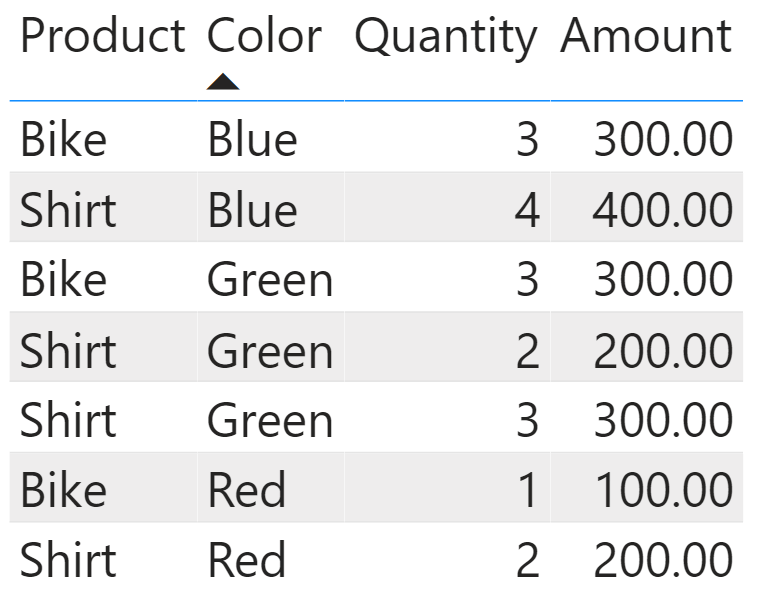On this simple table, we run a SUMMARIZE query that works just as expected:

```EVALUATE
SUMMARIZE (
Sales,
Sales[Color],
"Sales", SUM ( Sales[Amount] )
)
```

This query produces the sales amount by color.Intuitively, we might think that SUMMARIZE started by grouping Sales by Sales[Color]; that it then iterated the result by computing the sum of Amount for all the rows with the same color, by creating a filter context over the color. Unfortunately, this would be an approximation of the steps performed by SUMMARIZE. What it does is more complex.

Because the query needs to group by color, SUMMARIZE splits the table in partitions – one for each color. This operation is called clustering. Clustering is the creation of partitions based on the columns used to group by. SUMMARIZE first clusters the table based on the color, and then computes the expression for each cluster by creating a filter context that limits the calculation to the iterated cluster. Because we are grouping by Sales[Color], SUMMARIZE splits the Sales table into three clusters based on the color.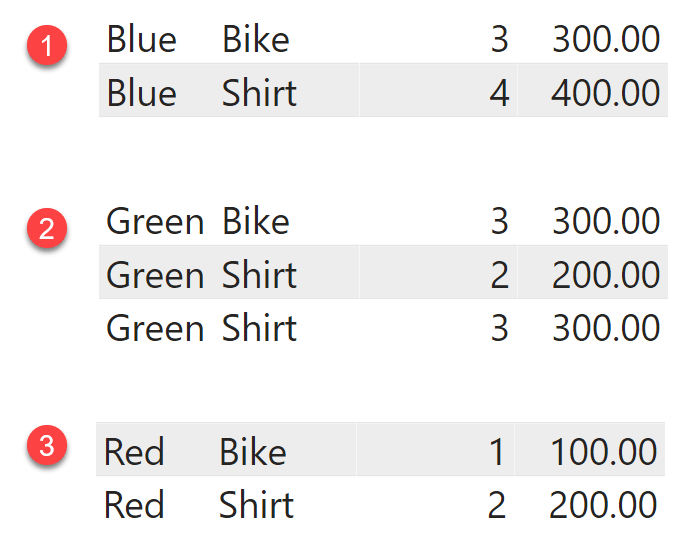Because we grouped by color, each cluster is identified by one color. In our scenario, Sales[Color] is the cluster header. The cluster header is the set of columns used in the groupby section of SUMMARIZE. The cluster header can contain multiple columns, even though in this first scenario we have only one column.

Once the clusters are ready, SUMMARIZE computes the value of SUM ( Sales[Amount] ) for the three clusters. Here is the first of the many shenanigans SUMMARIZE tends to engage in: to restrict the calculation to an individual cluster, SUMMARIZE does not create a filter context containing the cluster header only. Instead, it creates a filter context using all the columns in the cluster, filtering the values that are present in the cluster.

For the Blue cluster we have three bikes for 300.00 USD and four shirts accounting for 400.00 USD. Therefore, the expression computed for the Blue cluster looks like this:

```EVALUATE
{
CALCULATE (
SUM ( Sales[Amount] ),
FILTER (
ALL ( Sales ),
( Sales[Color], Sales[Product], Sales[Quantity], Sales[Amount] )
IN {
( "Blue", "Bike", 3, 300 ),
( "Blue", "Shirt", 4, 400 )
}
)
)
}
```

The expression filters all the columns, and the requirement is that the value of all the columns belongs to a row in the cluster. You can double-check this behavior by running the following query, as a test:

```EVALUATE
SUMMARIZE (
Sales,
Sales[Color],
"Test", ISFILTERED ( Sales[Quantity] )
)
```

The query returns TRUE in Test. This indicates that Sales[Quantity] is being actively filtered, even though it does not appear anywhere in the groupby columns. Indeed, Sales[Quantity] is filtered inside an expression computed by SUMMARIZE, because Sales[Quantity] is one of the columns of the clusters created to slice by color. Although the net effect looks the same, it is not. While it is true that each cluster filters only one color, the cluster is actually filtering much more than just the color.

Let us see why this is relevant. Try to guess the result of the following query, before moving forward:

```EVALUATE
SUMMARIZE (
Sales,
Sales[Color],
"Sales", SUM ( Sales[Amount] ),
"All Sales",
CALCULATE (
SUM ( Sales[Amount] ),
REMOVEFILTERS ( Sales[Color] )
)
)
```

Let me be very clear: if I were to guess, based on common sense, I would have said that All Sales returns the sales of all products. Instead, the result of All Sales is not the grand total of sales; it is quite a strange number that you can understand only by understanding clustering.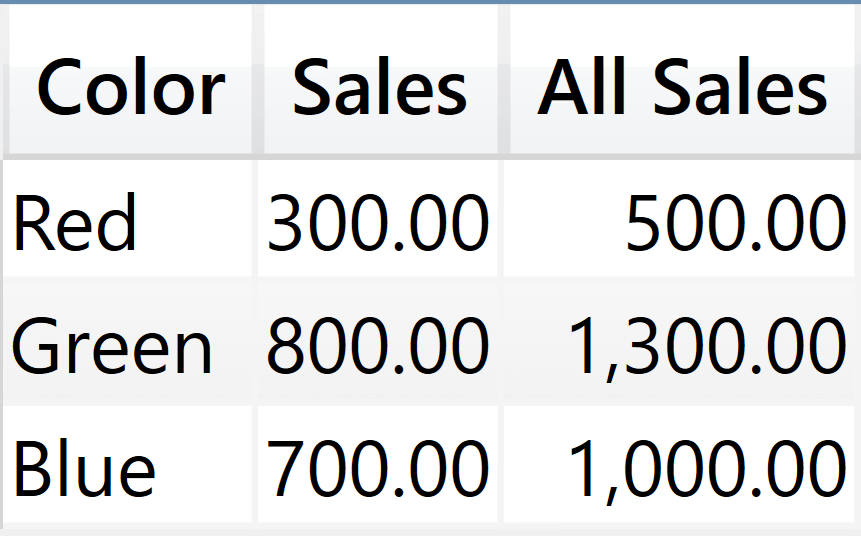If you rely on the intuitive behavior, you would assume that REMOVEFILTERS ( Sales[Color] ) removes the filter from the Sales[Color] column, thus making all rows of Sales visible. Indeed, REMOVEFILTERS removes the filter from Sales[Color] but it does not remove the filter from all the other columns in the cluster. Therefore, the evaluation of All Sales for the Blue cluster is the following:

```EVALUATE
{
CALCULATE (
CALCULATE (
SUM ( Sales[Amount] ),
REMOVEFILTERS ( Sales[Color] )
),
FILTER (
ALL ( Sales ),
( Sales[Color], Sales[Product], Sales[Quantity], Sales[Amount] )
IN {
( "Blue", "Bike", 3, 300 ),
( "Blue", "Shirt", 4, 400 )
}
)
)
}
```

The inner CALCULATE removes the filter on Sales[Color], but it does not modify all the remaining filters on Sales[Product], Sales[Quantity], and Sales[Amount]. If you remove the filter on Sales[Color] and you keep the filters on the other columns, then the combination (Green, Bike, 3, 300) is the only additional row that becomes visible in the filter context.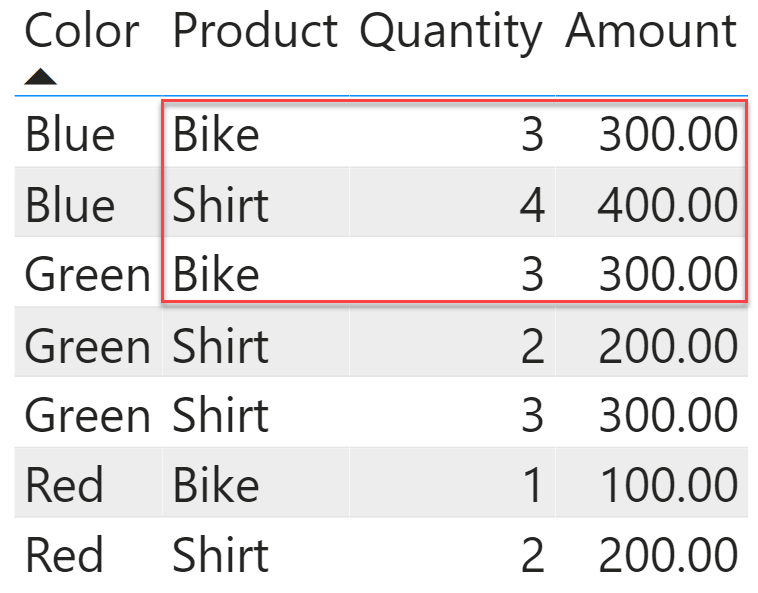The columns that are present in each cluster depend on the table that you use as the starting point for SUMMARIZE. Indeed, even though we usually SUMMARIZE a table in the model, SUMMARIZE can actually group any table expression. You have full control over this. For example, we can alter the query slightly to obtain a variant. Instead of summarizing Sales, we summarize ALL ( Sales[Color], Sales[Quantity] ):

```EVALUATE
SUMMARIZE (
ALL ( Sales[Color], Sales[Quantity] ),
Sales[Color],
"Sales", SUM ( Sales[Amount] ),
"All Sales",
CALCULATE (
SUM ( Sales[Amount] ),
REMOVEFILTERS ( Sales[Color] )
)
)
```

Despite looking unusual, this query runs just fine. It creates clusters with two columns – Sales[Color] and Sales[Quantity]) – and then it produces this result.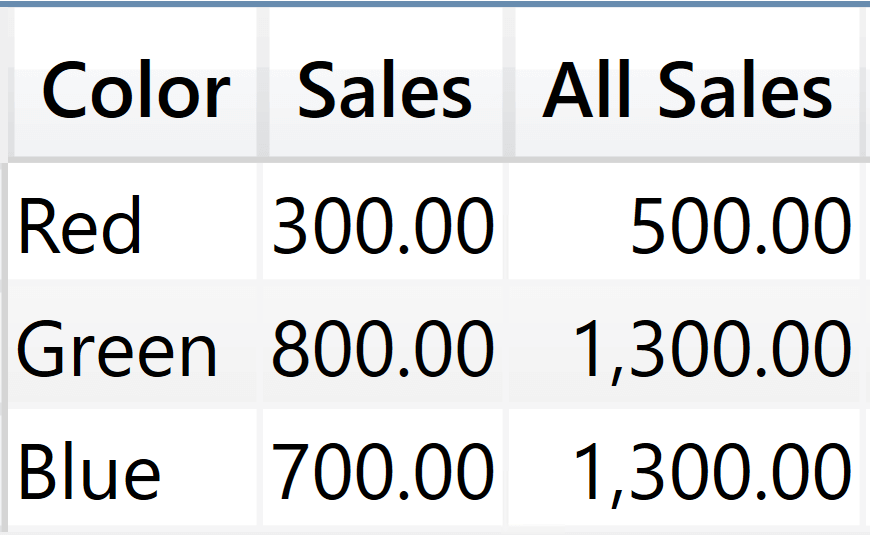Let us focus on the Blue cluster again. Where does 1,300 comes from? In order to understand it we first build the cluster, noting that there are now only two columns in the clusters: Sales[Color] and Sales[Quantity]. By removing the filter on Sales[Color], the only filter remaining is the one on Sales[Quantity] that filters two values: 3 and 4. Therefore, the result is the sum of Sales[Amount] for all the columns with a quantity of three or four:

```EVALUATE
{
CALCULATE (
CALCULATE (
SUM ( Sales[Amount] ),
REMOVEFILTERS ( Sales[Color] )
),
FILTER (
ALL ( Sales[Color], Sales[Quantity] ),
( Sales[Color], Sales[Quantity] )
IN {
( "Blue", 3 ),
( "Blue", 4 )
}
)
)
}
```

Looking at this latter code, it is important that the FILTER – introduced to restrict the cluster – be now working only on Sales[Color] and Sales[Quantity]. If you erroneously write code that filters the entire table, you would not produce the result of SUMMARIZE. Instead, the following query returns 1,000:

```EVALUATE
{
CALCULATE (
CALCULATE (
SUM ( Sales[Amount] ),
REMOVEFILTERS ( Sales[Color] )
),
FILTER (
ALL ( Sales ),
( Sales[Color], Sales[Quantity] )
IN {
( "Blue", 3 ),
( "Blue", 4 )
}
)
)
}
```

Indeed, in this last expression the filter on Sales of the outer CALCULATE reintroduces a filter context on all the columns of the table, producing again 1,000 instead of 1,300. Remember that this code is useful to understand what SUMMARIZE does: we need to write it correctly to produce the same output as what SUMMARIZE would.

## Why does SUMMARIZE use clustering?

At this point, it is a logical question: why does SUMMARIZE produce such a complex flow, when it could just use the cluster headers to filter the table? The reason is that SUMMARIZE is more powerful than what we typically use it for. SUMMARIZE can group by any column in the source table, even extension columns added through calculations.

For example, look at this query:

```EVALUATE
SUMMARIZE (
Sales,
"@Large Sale", IF ( Sales[Quantity] > 2, "Large", "Small" )
),
[@Large Sale],
"Amt", SUM ( Sales[Amount] )
)
```

The query works and it slices the Amt calculation values by the newly introduced column.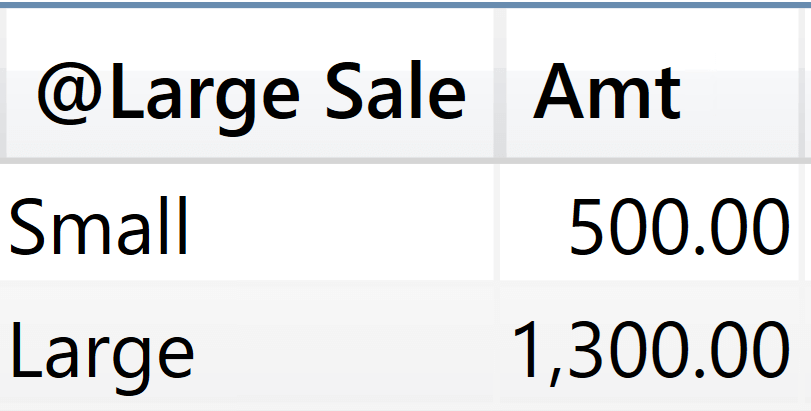The [@Large Sale] column does not belong to the model. It is a calculated column computed by ADDCOLUMNS in the query. As a calculated column, it does not have a model lineage. Therefore, it is impossible to filter the model based on [@Large Sale]. SUMMARIZE does not rely on a filter on [@Large Sale]. Instead, it considers [@Large Sale] as a cluster header. Each value of [@Large Sale] is associated to the set of values in all the other columns of Sales for the rows that are in the same cluster. SUMMARIZE uses the filter on all the columns in the cluster that produced a given value for [@Large Sale] to compute the value of Amt. In other words, in order to group by [@Large Sale], SUMMARIZE produces a filter that filters all the columns in Sales. For example, the filter for Small is created using the values of all the rows and columns of Sales that belong to the cluster whose header is Small.

We can double-check this behavior by creating a table that contains only the [@Large Sale] column. We do this by using SELECTCOLUMNS:

```EVALUATE
SUMMARIZE (
SELECTCOLUMNS (
Sales,
"@Large Sale", IF ( Sales[Quantity] > 2, "Large", "Small" )
),
"@Large Sale", [@Large Sale]
),
[@Large Sale],
"Amt", SUM ( Sales[Amount] )
)
```

In this query, the clusters contain only one column that does not have any lineage. Therefore, the filter context generated by SUMMARIZE is not effective against Sales, which in turn produces the same grand total result on every row.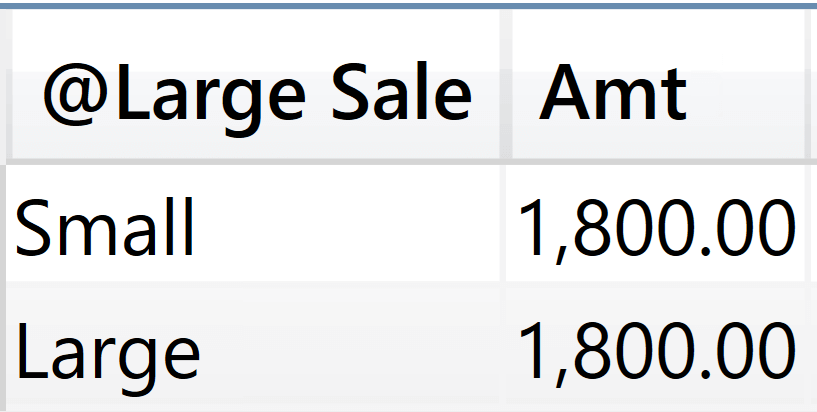In most scenarios, if your calculations follow the rules, SUMMARIZE gives you the opportunity to perform grouping based on based on columns that are not originating from the model .

Though extremely powerful, this behavior is very seldom used. The reason is that the mechanism to implement the grouping by extension columns is rather complex, as we are learning. It being so complex, we usually avoid using it and we rely on different grouping techniques. For instance, the GROUPBY function makes clustering more evident by using the CURRENTGROUP function. Despite being less powerful, it gained more popularity because it is more intuitive.

## Clustering and expanded tables

Now that we have introduced the concept of clustering, we can move one step further and discuss the impact of expanded tables on clustering. SUMMARIZE can use as a cluster header any column of the expanded table you use as the first argument. So far we have oftentimes given a simplified description, by saying that SUMMARIZE can group by any related column. The correct description is that SUMMARIZE can cluster by any column of the expanded table. Because table expansion goes from the many-side to the one-side of a regular relationship, it naturally follows that SUMMARIZE can group by any column of a related table joined many-to-one with a regular relationship to the base table.

Consequently, SUMMARIZE does not work across limited relationships, including both many-to-many cross-filter relationships and cross-island relationships.

Moreover, there is another important consequence to this: the cluster contains all the columns of the expanded table, not only the columns from the base table. It is advised to choose the base table wisely in order to maximize your chances of obtaining the result you want. Let us elaborate on this with a different model.

Let us work on a slightly different model: we add a Product table and replace the product columns in Sales with a Product Code column.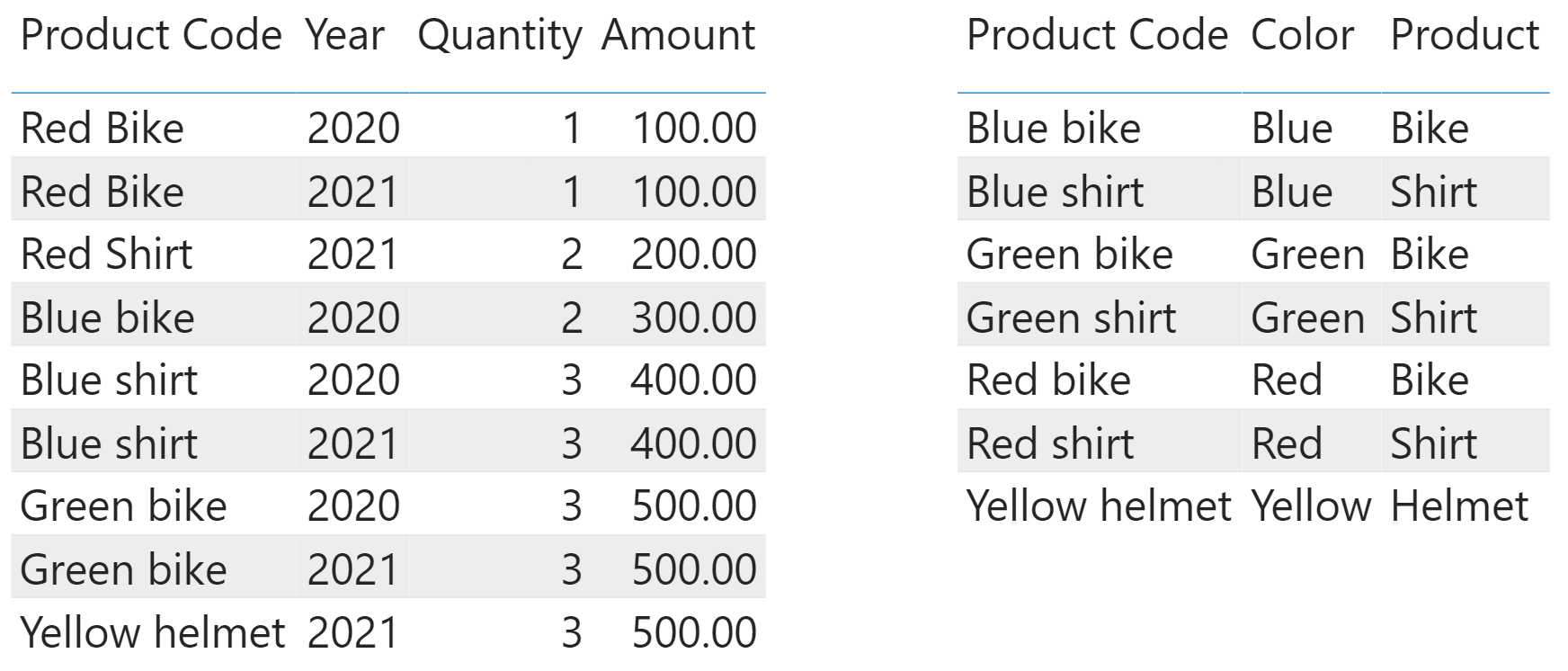With the knowledge we have gained so far, it is now easy to understand why the following query produces an unexpected result:

```EVALUATE
SUMMARIZE (
Sales,
'Product'[Color],
"Sales", SUM ( Sales[Amount] ),
"All Sales",
CALCULATE (
SUM ( Sales[Amount] ),
REMOVEFILTERS ( 'Product'[Color] )
)
)
```

The cluster header contains Product[Color], but the cluster filter contains all the columns in the expanded table Sales. This includes Product Code, Year, Quantity, and Amount from Sales, plus all the columns of Product. Therefore, despite the removal of the filter from Product[Color] by REMOVEFILTERS, both Sales and All Sales calculations produce the same result.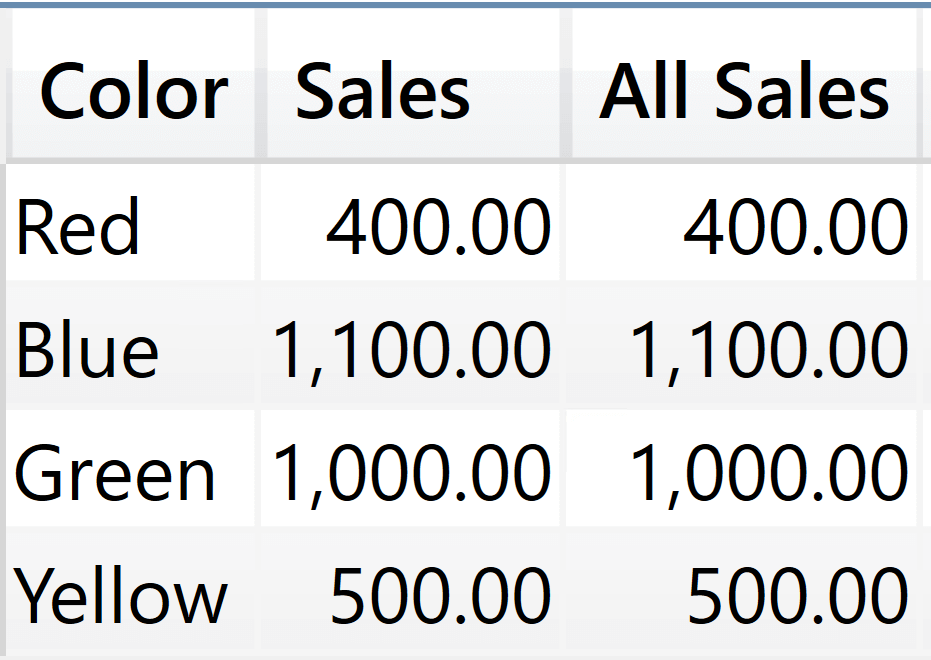If you expand REMOVEFILTERS to remove any filter from the Product table, you still obtain the same result:

```EVALUATE
SUMMARIZE (
Sales,
'Product'[Color],
"Sales", SUM ( Sales[Amount] ),
"All Sales",
CALCULATE (
SUM ( Sales[Amount] ),
REMOVEFILTERS ( 'Product' )
)
)
```

The reason is that the clusters contain all the columns in Sales, including Sales[Product Code]. No matter how many filters you remove from the cluster header, the real filter is coming from the other columns in Sales. Besides, we are using a model with only two tables. When the model contains tens of tables, as is the case for most real-world data models, the expanded version of the Sales table is likely to include an humongous amount of columns.

This impacts not only the semantics: it becomes harder and harder to figure out where the filter is being applied. It also has a strong impact on performance. Indeed, just by using SUMMARIZE you are dealing with gigantic filters on a huge number of columns. This typically results in very large materialization, hence in poor performance.

A common question among DAX newbies is what the difference is between using SUMMARIZE on the Sales table or on the Product table. There are multiple differences.

First, the size of the clusters is very different. The expanded Sales table is much larger than the expanded Product table, which mostly expands to itself only.

Moreover, by using SUMMARIZE over Sales, the clusters contain only the existing combinations of values present in Sales. A product that has never been sold does not appear, because there are no corresponding transactions for it in Sales. As a result, it is filtered out from the result.

The first point is important. By using the smallest possible table for the creation of the clusters, you not only obtain better performance – you also obtain a result that is much easier to understand because the cluster is less wide.

Still, using a narrower set for the clusters does not automagically solve all the problems of SUMMARIZE. As an exercise, figure out by yourself the reason why the next query produces its result. First, the query:

```EVALUATE
SUMMARIZE (
Product,
'Product'[Color],
"Sales", SUM ( Sales[Amount] ),
"All Colors",
CALCULATE (
SUM ( Sales[Amount] ),
REMOVEFILTERS ( 'Product'[Color] )
),
"All Sales",
CALCULATE (
SUM ( Sales[Amount] ),
REMOVEFILTERS ( 'Product' )
)
)
```

And then the result.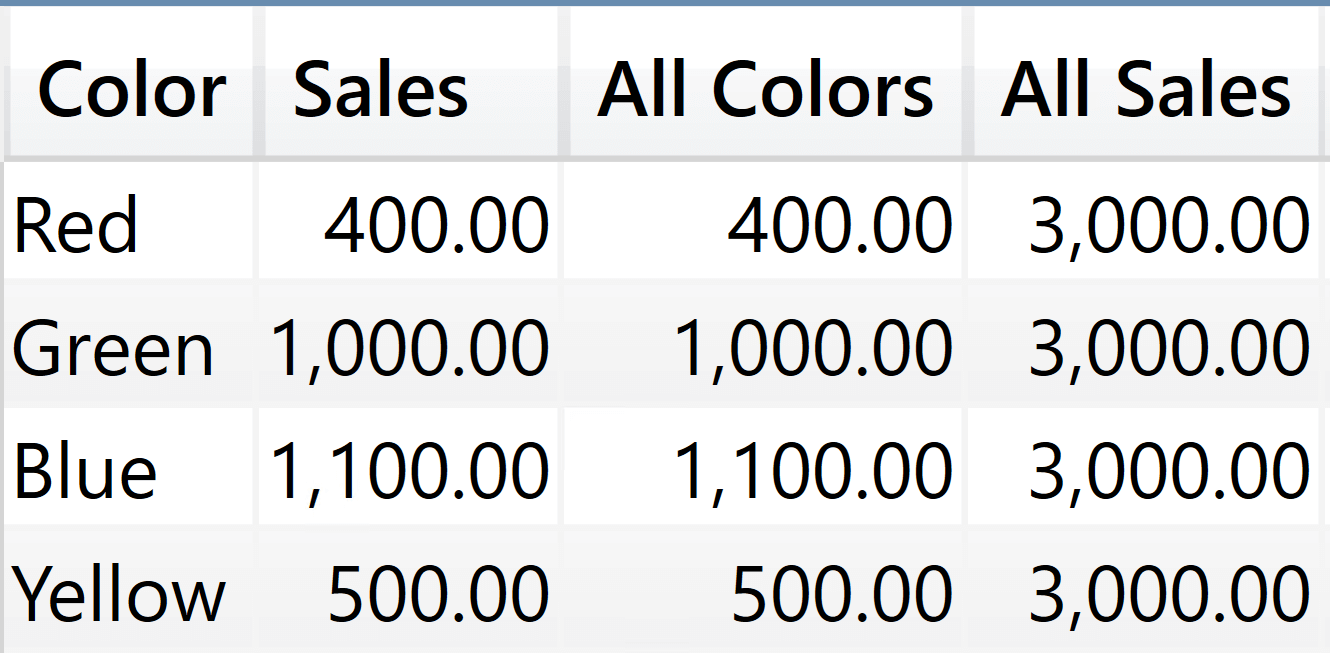All Colors removes the filter from Product[Color], but it does not remove the filter from all the other columns in Product. As such, it keeps the filter on the Product[Product Code] column, which uniquely identifies a product. Therefore, the Red cluster still contains only red products, even though we have removed the filter on Product[Color].

All Sales removes any filters from Product: This way, we remove all the filters from the columns in the cluster, obtaining the expected result.

## Taming the SUMMARIZE beast

One step at a time, we are figuring out how SUMMARIZE works. By now, you should be familiar with the idea of clustering. Once you have digested the concept of clustering, you are probably able to better understand why we consider SUMMARIZE to be a dangerous function. This is not to say that you cannot use SUMMARIZE, or that it does not work. It works, but what it does is not what you think it should.

The easiest way to reduce the complexity of SUMMARIZE is to reduce the number of columns in the cluster so that it contains only the cluster header columns. You can achieve this result in multiple ways. One way – a bit counterintuitive – is to nest multiple SUMMARIZE functions. Look at the following query, where the outer SUMMARIZE is using an inner SUMMARIZE as the base table:

```EVALUATE
SUMMARIZE (
SUMMARIZE ( Sales, 'Product'[Color] ),
'Product'[Color],
"Sales", SUM ( Sales[Amount] ),
"All Colors",
CALCULATE (
SUM ( Sales[Amount] ),
REMOVEFILTERS ( 'Product'[Color] )
),
"All Sales",
CALCULATE (
SUM ( Sales[Amount] ),
REMOVEFILTERS ( 'Product' )
)
)
```

This query produces the expected result in both columns.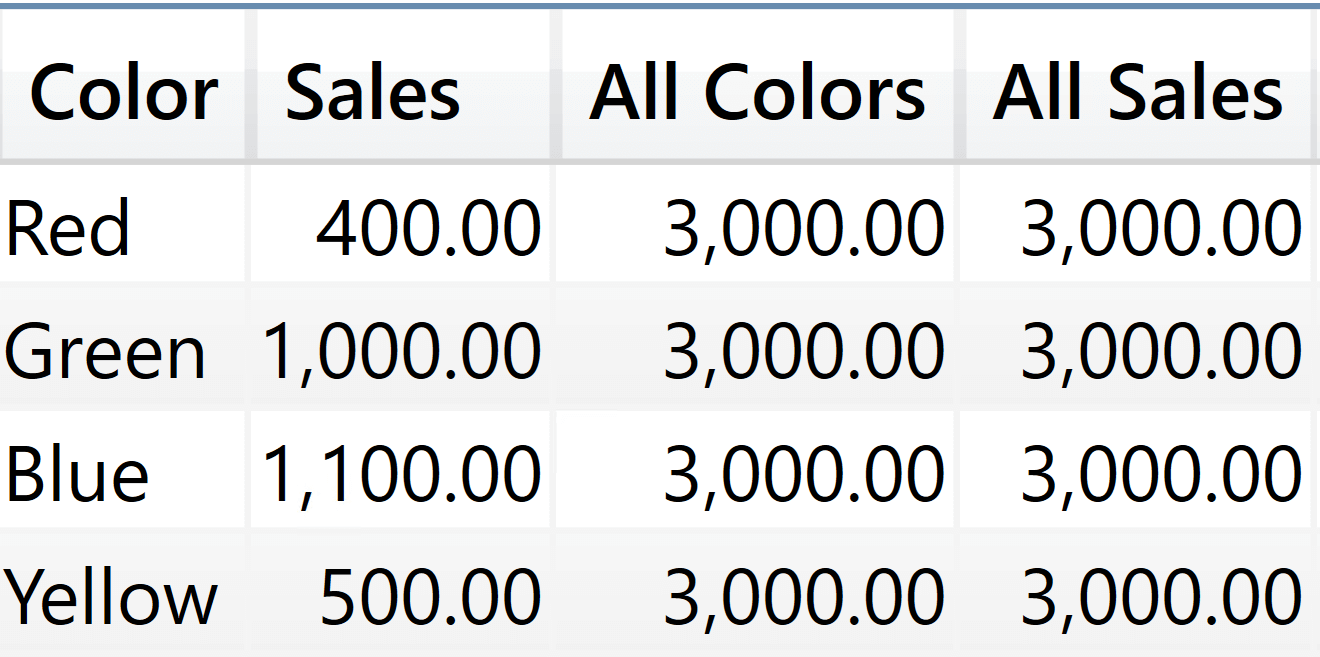The reason is quite simple: the inner SUMMARIZE generates a table that contains only the Product[Color] column. Therefore, the clusters contain only the Product[Color] column and when we remove the filter from Product[Color], we are actually modifying the intuitive filter context.

This last consideration leads to the code that we suggest as a best practice: do not use SUMMARIZE to generate new columns, use it only to perform grouping. Indeed, in our last query we can easily swap the outer SUMMARIZE with a more intuitive ADDCOLUMNS whose semantics are much simpler. We only need to add CALCULATE in the first extension column, because ADDCOLUMNS does not generate a filter context; it only relies on the row context:

```EVALUATE
SUMMARIZE ( Sales, 'Product'[Color] ),
"Sales", CALCULATE ( SUM ( Sales[Amount] ) ),
"All Colors",
CALCULATE (
SUM ( Sales[Amount] ),
REMOVEFILTERS ( 'Product'[Color] )
),
"All Sales",
CALCULATE (
SUM ( Sales[Amount] ),
REMOVEFILTERS ( 'Product' )
)
)
```

## Row context and filter context

Another aspect of SUMMARIZE is that it is the only function in DAX that creates both a row context and a filter context. During the evaluation of the new columns, SUMMARIZE iterates on the clusters and it also generates:

• A row context, containing the cluster headers;
• A filter context, containing all the columns in the cluster including the cluster headers.

This unique behavior adds a bit more confusion to a function that is already complex by nature. Indeed, the following query produces a list of colors, duplicating the same color name in all the columns:

```EVALUATE
SUMMARIZE (
Sales,
'Product'[Color],
"Color 1", Product[Color],
"Color 2", SELECTEDVALUE ( Product[Color] ),
"Color 3", VALUES ( Product[Color] )
)
```

As you see, the color is repeated in the Color 1, Color 2, and Color 3 columns.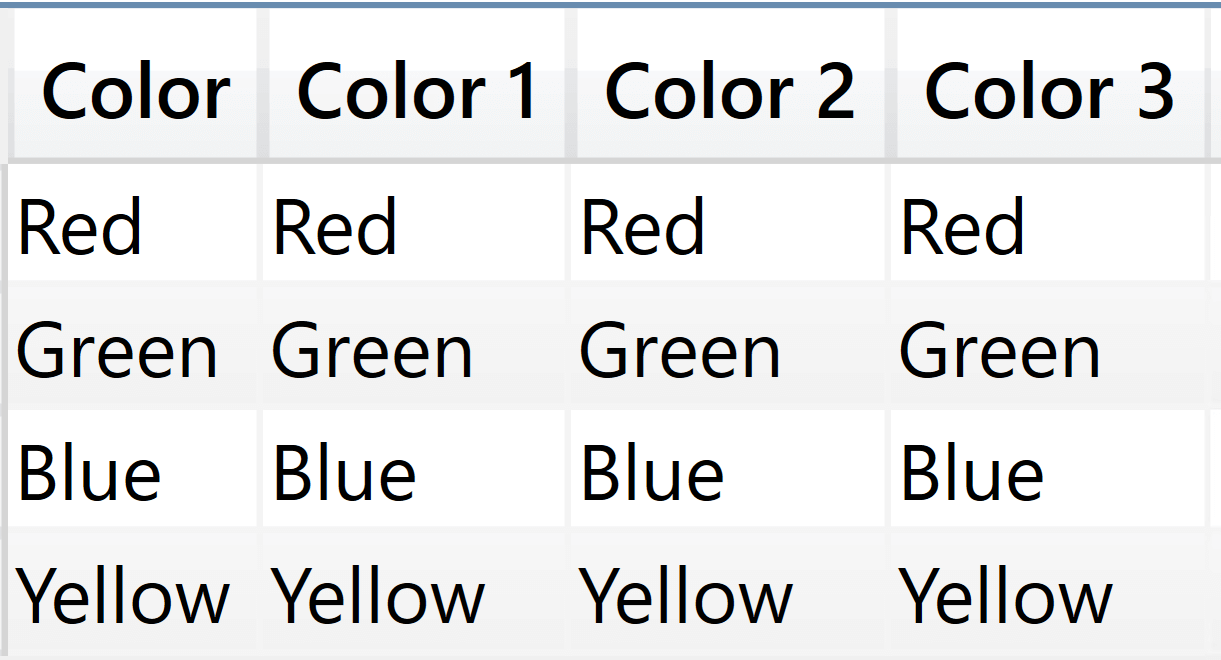Color 1 is based on the row context, which contains only the cluster headers. Color 2 and Color 3 rely on the filter context.

In itself, this behavior is not bad. It only creates some confusion because when you use CALCULATE inside SUMMARIZE, you not only change the filter context, you also invoke a context transition on all the columns in the cluster header.

SUMMARIZE needs to iterate over the cluster to let developers use ALLSELECTED inside the code of columns computed by that SUMMARIZE. Remember that ALLSELECTED relies on the presence of shadow filter contexts to work properly. If you are not familiar with ALLSELECTED and shadow filter contexts, you can find more information here: The definitive guide to ALLSELECTED – SQLBI.

As we said, the dual nature of SUMMARIZE, both as an iterator over the cluster headers and a generator of filter contexts on the cluster content, is needed to obtain what you expect out of ALLSELECTED. Indeed, by iterating over the cluster headers, SUMMARIZE generates a shadow filter context on the cluster headers. That way, invoking ALLSELECTED inside SUMMARIZE restores the filter context on the cluster headers, obtaining the result you want.

Using ADDCOLUMNS instead of SUMMARIZE does not change this behavior. ADDCOLUMNS iterates on the table returned by SUMMARIZE and it also generates a shadow filter context. The advantage of using ADDCOLUMNS instead of SUMMARIZE to add columns is that we are more accustomed to the shadow filter context being created by iterators – and when we think about SUMMARIZE we do not picture an iterator. Indeed, SUMMARIZE is an iterator: it iterates the clusters.

## How do we know all this stuff?

I know, you think we know everything about DAX. That is not true. We do know a lot, but like anyone else we deal with many doubts and questions. You learn by reading our articles, we learn by talking and meeting with the creators of DAX.This picture dates back to November 2014. On the floor in Seattle, I was asking Jeffrey Wang how on earth SUMMARIZE worked. It was 6PM and Jeffrey, instead of just smiling and going home at the end of a working day, sat down with me and patiently explained the details to me.

Since then, it has taken me another six years to completely understand the details. I have written several articles about SUMMARIZE, this being the latest one with the most comprehensive understanding I have so far about this function.

## Conclusions

As you have seen, SUMMARIZE comes with a high level of complexity. It is an extremely powerful function. Its ability to group by columns without a proper model lineage is somewhat unique. You should not take SUMMARIZE lightly. As long as you use SUMMARIZE for its primary goal, which is grouping a table by a related column, everything works fine. As soon as you make SUMMARIZE compute new columns, you are putting yourself at risk of obtaining inaccurate results.

There are few scenarios, if any, where SUMMARIZE is actually required in order to compute new columns. In almost all the scenarios we have ever faced, using ADDCOLUMNS results in simpler, cleaner and more efficient code.

SUMMARIZECreates a summary of the input table grouped by the specified columns.

`SUMMARIZE ( <Table> [, <GroupBy_ColumnName> [, [<Name>] [, [<Expression>] [, <GroupBy_ColumnName> [, [<Name>] [, [<Expression>] [, … ] ] ] ] ] ] ] )`

ADDCOLUMNSReturns a table with new columns specified by the DAX expressions.

`ADDCOLUMNS ( <Table>, <Name>, <Expression> [, <Name>, <Expression> [, … ] ] )`

SUMMARIZECOLUMNSCreate a summary table for the requested totals over set of groups.

`SUMMARIZECOLUMNS ( [<GroupBy_ColumnName> [, [<FilterTable>] [, [<Name>] [, [<Expression>] [, <GroupBy_ColumnName> [, [<FilterTable>] [, [<Name>] [, [<Expression>] [, … ] ] ] ] ] ] ] ] ] )`

SUMAdds all the numbers in a column.

`SUM ( <ColumnName> )`

REMOVEFILTERSCALCULATE modifier

Clear filters from the specified tables or columns.

`REMOVEFILTERS ( [<TableNameOrColumnName>] [, <ColumnName> [, <ColumnName> [, … ] ] ] )`

CALCULATEContext transition

Evaluates an expression in a context modified by filters.

`CALCULATE ( <Expression> [, <Filter> [, <Filter> [, … ] ] ] )`

ALLCALCULATE modifier

Returns all the rows in a table, or all the values in a column, ignoring any filters that might have been applied.

`ALL ( [<TableNameOrColumnName>] [, <ColumnName> [, <ColumnName> [, … ] ] ] )`

FILTERReturns a table that has been filtered.

`FILTER ( <Table>, <FilterExpression> )`

SELECTCOLUMNSReturns a table with selected columns from the table and new columns specified by the DAX expressions.

`SELECTCOLUMNS ( <Table> [[, <Name>], <Expression> [[, <Name>], <Expression> [, … ] ] ] )`

GROUPBYCreates a summary the input table grouped by the specified columns.

`GROUPBY ( <Table> [, <GroupBy_ColumnName> [, [<Name>] [, [<Expression>] [, <GroupBy_ColumnName> [, [<Name>] [, [<Expression>] [, … ] ] ] ] ] ] ] )`

CURRENTGROUPAccess to the (sub)table representing current group in GroupBy function. Can be used only inside GroupBy function.

`CURRENTGROUP ( )`

ALLSELECTEDCALCULATE modifier

Returns all the rows in a table, or all the values in a column, ignoring any filters that might have been applied inside the query, but keeping filters that come from outside.

`ALLSELECTED ( [<TableNameOrColumnName>] [, <ColumnName> [, <ColumnName> [, … ] ] ] )`